## Range Prediction

The range of the aircraft is a function of the rate at which fuel is being burnt, the duration of cruise, the flight speed and the aerodynamic performance during the flight. The mode of flight can also have a measurable effect on the distance traveled. Of major importance is the fact that the fuel used to supply the engines must be carried onboard the aircraft. This fuel weight must be supported by the aerodynamic lift along with the aircraft structural weight and the payload. While the structure and payload weights are fixed, the fuel weight changes significantly during the flight, leading to changing performance during long range cruise.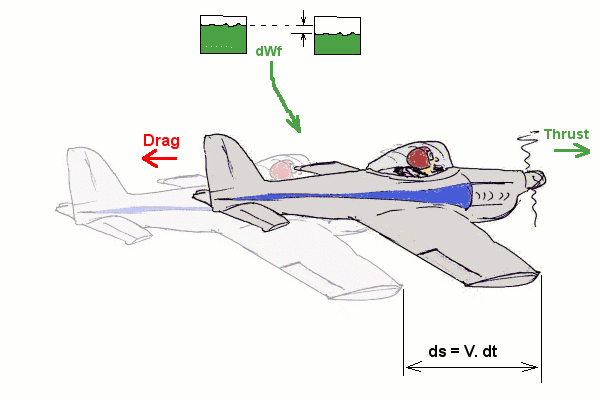A simple method of analyzing the aircraft cruise performance, during level flight, is to look at the balance of energy being created by the combustion of fuel and the energy required to overcome the resistance to motion through the air.

For a short period of time, dt, while the aircraft moves a distance, ds, at a velocity V,
that is, ds = V.dt, the energy components are;

(1a) Energy obtained from fuel burnt = dWf.Hc.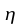where, dWf is weight of fuel burnt during time dt, Hc is energy content (calorific value) of fuel andis the efficiency of the propulsion system.

This is also equivalent to,

(1b) Energy produced by the propulsion system = T.V.dt

where, T is the thrust produced, V is the flight velocity, hence T.V is the supplied power over time dt.

Thus T.V.dt = Hc..dWf or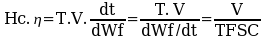where TSFC, thrust specific fuel consumption,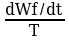, is a more easily obtainable measure of engine performance.

(2) Energy dissipated due to aircraft motion = D.V. dt

where D is drag on the vehicle, V is flight velocity,hence D.V is required power to be overcome during time dt.

The balance of energy components gives,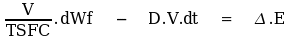.

In terms of specific energy,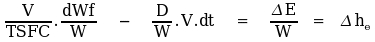.

Rearranging gives,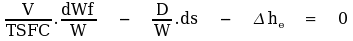.

Based on the assumption of level flight, L = W, and the assumption of roughly constant altitude and velocity cruise,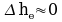, plus the substitution for change in weight in terms of loss of vehicle weight rather than fuel burn weight,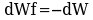, then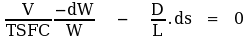or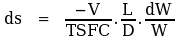..... (1)

Again assuming approximate steady conditions where velocity and propulsion performance is kept constant and that the aerodynamic parameter L/D is maintained at a constant value then integration of this equation gives a range of,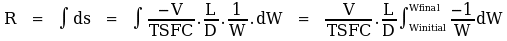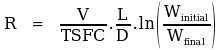..... (2).

Equation (2) is the classic Breuget Range equation. It can be used to give reasonable estimates of range in still air. However the assumption of constant L/D and constant V/TSFC may not be too accurate in practice. To maintain constant L/D with the changing weight the aircraft would need to drift up in altitude so that a constant angle of attack was maintained. It may also be required that the aircraft change speed to maintain a constant V/TSFC. So to get detailed estimates over long range cruise conditions it may be necessary to do a numerical summation of the short segments where the assumptions are accurate.

## Range with Headwind or Tailwind.

The above calculations are based on still air conditions. If the aircraft is flying into a headwind or with a tailwind, then the distance travelled through the air is the same but the ground distance covered will change.

The energy dissipated in overcoming drag is unchanged but the effective energy output relative to the ground will be based on the relative velocity, (V - Vw) where Vw is the velocity of the wind, positive meaning a headwind.

The energy balance becomes,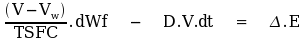and the final range equation becomes,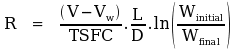## Endurance

This is the total time taken during flight. It is directly related to the rate of fuel consumption.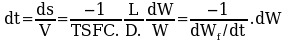If the aircraft is flown using a constant fuel flow rate.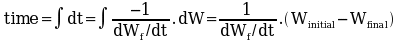## Optimums

In order to maximise range for a given load of fuel, a balance of V/TSFC and L/D must be found. Increasing V will increase range up to a point where the increasing drag will start to reduce L/D so that the range, which is the product of these two terms, again starts to reduce.

In order to maximise endurance, fuel flow rate must be reduced. For engines with roughly constant TSFC this means reducing thrust by flying slowly at minimum drag speed.

The optimum flight speed for maximum endurance will be quite low and may be approaching the stall speed, whereas the optimum flight speed for range will be high.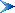Table of Contents

© AMME, University of Sydney, 1998-2006.